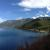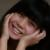PHP form 表单 动态输出 解决方案 ,求交流和分享

年薪达不到25W大数据工程师、拿不到Offer全额退款->>><input type="text" name="name1"  value="<?php echo \$foo['name1']; ?>"/>

<select name="name2">
<option value="0"<?php \$options->es('name2','0');?>>否</option>
<option value="1"<?php \$options->es('name2','1');?>>是</option>
</select>

0<select name="name2" id="name2">
<option value="0">否</option>
<option value="1">是</option>
</select>
<script type="text/javascript">
document.getElementById("name2").value = '<?php echo \$val ?>';
</script>

0<?php
/**
* 表单解释类
* @author php-朱磊
*
*/
class HtmlForm extends Html {

/**
* 生成checkbox
* @param \$array name:chekcbox名称.options:选项.selected:选中数组.gule:间隔,默认&nbsp;
* @param \$params
*/
public function checkbox(\$array,\$params=null){
\$name=\$array['name'];
\$chkecboxArr=\$array['options'];
\$selected=(array)\$array['selected'];
\$gule=\$array['gule']?\$array['gule']:'&nbsp;';
\$checkboxs=array();
if (!is_null(\$params)){//附加属性
\$appendParams='';
foreach (\$params as \$key=>\$val){
\$appendParams.=" {\$key}='{\$val}' ";
}
}
foreach (\$chkecboxArr as \$key=>\$val){
\$isSelected=in_array(\$key,\$selected)?"checked='checked'":'';
\$checkboxs[]="<label><input type='checkbox' name='{\$name}[]' value='{\$key}' {\$isSelected} {\$appendParams} />{\$val}</label>";
}
return implode(\$gule,\$checkboxs);
}

/**
* 生成checkbox
* @param \$array name:chekcbox名称.options:选项,多维数组.selected:选中数组.gule:间隔,默认&nbsp;
* @param \$params
*/
public function checkboxTwo(\$array,\$params=null){
\$name=\$array['name'];
\$chkecboxArr=\$array['options'];
\$selected=(array)\$array['selected'];
\$checkboxKey=\$array['key'];
\$checkboxValue=\$array['value'];
\$gule=\$array['gule']?\$array['gule']:'&nbsp;';
\$checkboxs=array();
if (!is_null(\$params)){//附加属性
\$appendParams='';
foreach (\$params as \$key=>\$val){
\$appendParams.=" {\$key}='{\$val}' ";
}
}
foreach (\$chkecboxArr as \$val){
\$isSelected=in_array(\$val[\$checkboxKey],\$selected)?"checked='checked'":'';
\$checkboxs[]="<label><input type='checkbox' name='{\$name}[]' value='{\$val[\$checkboxKey]}' {\$isSelected} {\$appendParams} />{\$val[\$checkboxValue]}</label>";
}
return implode(\$gule,\$checkboxs);
}

/**
* @param \$array name:radio名称.options:选项.selected:选中数组.gule:间隔,默认&nbsp;
* @param \$params
*/
\$name=\$array['name'];
\$chkecboxArr=\$array['options'];
\$selected=\$array['selected'];
\$gule=\$array['gule']?\$array['gule']:'&nbsp;';
\$checkboxs=array();
if (!is_null(\$params)){//附加属性
\$appendParams='';
foreach (\$params as \$key=>\$val){
\$appendParams.=" {\$key}='{\$val}' ";
}
}
foreach (\$chkecboxArr as \$key=>\$val){
\$isSelected=((string)\$key===(string)\$selected)?"checked='checked'":'';
\$checkboxs[]="<label><input type='radio' name='{\$name}' value='{\$key}' {\$isSelected} {\$appendParams} />{\$val}</label>";
}
return implode(\$gule,\$checkboxs);
}

/**
* @param \$array name:chekcbox名称.options:选项,多维数组.selected:选中数组.gule:间隔,默认&nbsp;.key:value值.value:选项名称
* @param \$params
*/
\$name=\$array['name'];
\$chkecboxArr=\$array['options'];
\$selected=\$array['selected'];
\$checkboxKey=\$array['key'];
\$checkboxValue=\$array['value'];
\$gule=\$array['gule']?\$array['gule']:'&nbsp;';
\$checkboxs=array();
if (!is_null(\$params)){//附加属性
\$appendParams='';
foreach (\$params as \$key=>\$val){
\$appendParams.=" {\$key}='{\$val}' ";
}
}
foreach (\$chkecboxArr as \$val){
\$isSelected=((string)\$val[\$checkboxKey]===(string)\$selected)?'checked':'';
\$checkboxs[]="<label><input type='radio' name='{\$name}' value='{\$val[\$checkboxKey]}' {\$isSelected} {\$appendParams} />{\$val[\$checkboxValue]}</label>";
}
return implode(\$gule,\$checkboxs);
}

/**
* 生成option
* @param \$array
* @param \$params
*/
public function options(\$array){
\$selected=\$array['selected'];
\$options=array();
foreach (\$array['options'] as \$key=>\$val){
\$isSelected=((string)\$key===(string)\$selected)?"selected='selected'":'';
\$options[]="<option {\$isSelected} label='{\$val}' value='{\$key}'>{\$val}</option>";
}
return implode("\r\n",\$options);
}

/**
* 生成options
* @param \$array options:选项,多维数组.key:value值.value:选项名称
* @param \$params
*/
public function optionsTwo(\$array){
\$selected=\$array['selected'];
\$optionKey=\$array['key'];
\$optionValue=\$array['value'];
\$options=array();
foreach (\$array['options'] as \$key=>\$val){
\$isSelected=((string)\$val[\$optionKey]===(string)\$selected)?"selected='selected'":'';
\$options[]="<option {\$isSelected} label='{\$val[\$optionValue]}' value='{\$val[\$optionKey]}'>{\$val[\$optionValue]}</option>";
}
return implode("\r\n",\$options);
}

}